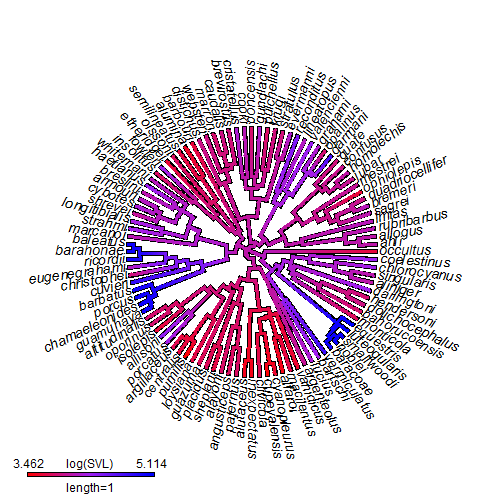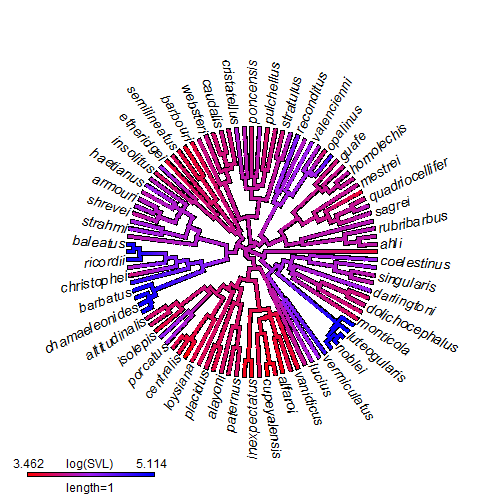## Tuesday, April 11, 2017

### Plotting only a subset of tip labels on a `type="fan"` style tree

Recently, responding to a user request, I demonstrated how to plot a tree showing text for only a subset of tip labels. The code I posted applied narrowly to the case of a right-facing tree; however we can apply more or less the same principle to plotting the tip labels on a fan-style tree. The only added complication is that for circular trees we also have to figure out how to rotate the text of the tip labels! Not only that, but by convention we want to avoid upside-down labels, no?

Here is a demo that uses the tree & data from Revell et al. (2015, Evolution). (Data available on Dryad here.) In the demo I use a `contMap` style plot, but the same idea could be used for any plotted fan tree style.

``````library(phytools)
row.names=1)
svl<-setNames(X[],rownames(X))[1:(nrow(X)-1)]
## create our "contMap" class object:
obj<-contMap(tree,svl,plot=FALSE)
## set desired colors
obj<-setMap(obj,c("red","purple","blue"))
## plot a test version with labels
plot(obj,leg.txt="log(SVL)",type="fan",legend=1,fsize=c(1.2,1),lwd=3)
````````````## now select every other tip label. These are the labels we will plot.
tips<-tree\$tip.label[seq(1,Ntip(tree),by=2)]
## get x & y limits for replotting
lastPP<-get("last_plot.phylo",envir=.PlotPhyloEnv)
xlim<-lastPP\$x.lim
ylim<-lastPP\$y.lim
plot(obj,xlim=xlim,ylim=ylim,ftype="off",leg.txt="log(SVL)",
type="fan",legend=1,lwd=3)
lastPP<-get("last_plot.phylo",envir=.PlotPhyloEnv)
xpos<-lastPP\$xx[sapply(tips,function(x,y) which(y==x),
y=obj\$tree\$tip.label)]
ypos<-lastPP\$yy[sapply(tips,function(x,y) which(y==x),
y=obj\$tree\$tip.label)]
for(i in 1:length(tips)){
angle<-atan(ypos[i]/xpos[i])*180/pi
if(xpos[i]<0) text(xpos[i],ypos[i],paste(gsub("_"," ",tips[i])," ",
sep=""),pos=2,font=3,srt=angle,offset=0,cex=1.2)
else text(xpos[i],ypos[i],paste(" ",gsub("_"," ",tips[i]),sep=""),
pos=4,font=3,srt=angle,offset=0,cex=1.2)
}
``````That's more or less the idea.